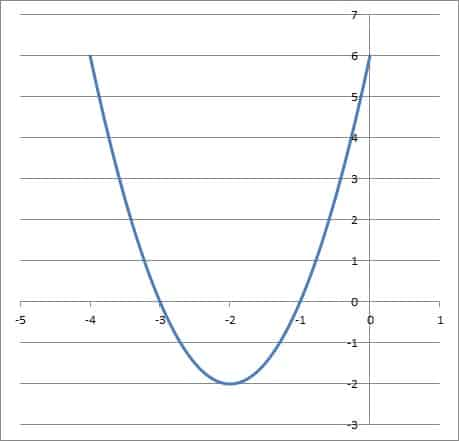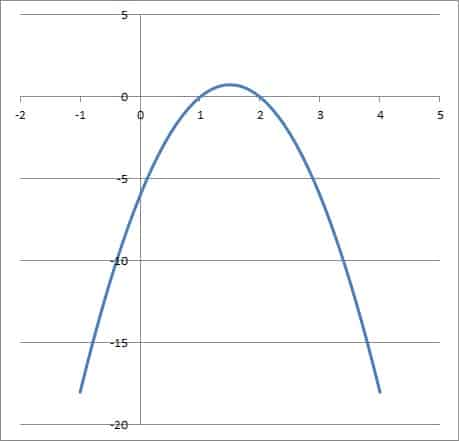# Questions About The Vertex Of A Parabola (5 Things To Know)

The vertex of a parabola is an extreme value of the function.  However, it helps to know when it is a maximum or minimum and when it might touch a coordinate axis.

So, what do you need to know about the vertex of a parabola?  A parabola’s vertex is the minimum value when a > 0, and in this case, the parabola is convex (concave up) and opens upward (like a cup shape). A parabola’s vertex is the maximum value when a < 0, and in this case, the parabola is concave (concave down) and opens downward (like a dome shape).

Of course, the vertex of a parabola can like on the x-axis, on the y-axis, and in some cases on both at the same time (that is, the vertex is at the origin).

In this article, we’ll answer some common questions about the vertex of a parabola and give some examples to make the concepts clear.

Let’s get started.

## Questions About The Vertex Of A Parabola

You might be familiar with what a parabola looks like, but you may still need to know when the vertex is a maximum or minimum and when it touches the x or y axes.

### Is The Vertex A Minimum Or Maximum?

The vertex of a parabola can be a maximum or minimum depending on the sign of the leading (quadratic) coefficient, which tells us the shape of the graph of a quadratic.

Here is how to tell if the vertex is a maximum or minimum of a parabola:

• Look at the leading coefficient (that is, the coefficient of the x2 term in the quadratic equation that the parabola comes from).
• Look at the concavity of the parabola (that is, examine the sign of the second derivative, f’’(x), of the quadratic function, f(x), that the parabola comes from).
• Look at the graph of the parabola (which way does it open: up or down?)

Let’s take a look at when each case occurs.

#### When Is The Vertex Of A Parabola The Minimum Point?

The vertex of a parabola is the minimum point when:

• The leading coefficient is positive (that is, in the quadratic equation y = ax2 + bx + c, we have a > 0).
• The parabola is convex, or concave up (that is, f’’(x) > 0, where f’’(x) is the second derivative of the quadratic function f(x) that the parabola comes from).
• The parabola opens upward (that is, the graph of the parabola is shaped like a cup, not a dome – it would hold water).
##### Example: The Vertex Of A Parabola Is The Minimum Point

• f(x) = 2x2 + 8x + 6

As you can see, the leading coefficient (of the x2 term) is a = 2.  Since 2 is positive (a > 0), we know that the vertex of the corresponding parabola is a minimum value.

We can also find the second derivative of the function:

• f’(x) = 4x + 8  [first derivative of f(x)]
• f’’(x) = 4  [second derivative of f(x)]

Since 4 is positive (f’’(x) > 0), we know that the function is convex (concave up).  This confirms that the vertex of the parabola is a minimum.

Finally, we can also visually confirm that the vertex is a minimum by examining the graph of the parabola below:The quadratic function f(x) = 2x2 + 8x + 6 is concave up, since a = 2 is positive. Thus, the vertex is a minimum of the function.

#### When Is The Vertex Of A Parabola The Maximum Point?

The vertex of a parabola is the maximum point when:

• The leading coefficient is negative (that is, in the quadratic equation y = ax2 + bx + c, we have a < 0).
• The parabola is concave, or concave down (that is, f’’(x) < 0, where f’’(x) is the second derivative of the quadratic function f(x) that the parabola comes from).
• The parabola opens downward (that is, the graph of the parabola is shaped like a dome, not a cup – water would run off the top).
##### Example: The Vertex Of A Parabola Is The Maximum Point

• f(x) = -3x2 + 9x – 6

As you can see, the leading coefficient (of the x2 term) is a = -3.  Since -3 is negative (a < 0), we know that the vertex of the corresponding parabola is a maximum value.

We can also find the second derivative of the function:

• f’(x) = -6x + 9  [first derivative of f(x)]
• f’’(x) = -6  [second derivative of f(x)]

Since -6 is negative (f’’(x) < 0), we know that the function is concave (concave down).  This confirms that the vertex of the parabola is a maximum.

Finally, we can also visually confirm that the vertex is a maximum by examining the graph of the parabola below:The quadratic function f(x) = -3x2 + 9x – 6 is concave down, since a = -3 is negative. Thus, the vertex is a maximum of the function.

### Can The Vertex Be The X-Intercept?

The vertex can be the x-intercept of a parabola in some cases (this happens when the vertex rests on the x-axis).  We can find out when this will occur by combining what we know about the x-intercept, vertex, and solutions of a quadratic function.

First, remember that the standard form of a quadratic function is given by:

• f(x) = ax2 + bx + c

or

• y = ax2 + bx + c

where a is not zero.

Also, remember that a vertex is unique – a parabola has only one vertex.  In this case, the vertex has a y-coordinate of zero, since it rests on the x-axis.

So, the corresponding quadratic equation y = ax2 + bx + c has one solution for y = 0.  This occurs when the discriminant is zero, or when b2 – 4ac = 0.

So, if the quadratic equation has b2 – 4ac = 0 (or b2 = 4ac), the corresponding parabola has its vertex on the x-axis (that is, the vertex is also the x-intercept).

#### Example: The Vertex Is The X-Intercept

• y = 3x2 + 18x + 27

We have a = 3, b = 18, and c = 27, which means:

• b2 – 4ac
• =182 – 4(3)(27)
• =324 – 324
• =0

Since the discriminant is zero, the corresponding parabola will have its vertex on the x-axis, as you can see in the graph below.

### Can The Vertex & Y-Intercept Be The Same?

The vertex can be the y-intercept of a parabola in some cases (this happens when the vertex rests on the y-axis).  We can find out when this will occur by combining what we know about the x-intercept, vertex, and solutions of a quadratic function.

First, remember that the standard form of a quadratic function is given by:

• f(x) = ax2 + bx + c

or

• y = ax2 + bx + c

where a is not zero.

The y-intercept occurs where x = 0.  This gives us

• y = ax2 + bx + c
• y = a(0)2 + b(0) + c
• y = c

In this case, the y-intercept is given by the point (x, y) = (0, c).

Recall that the vertex form of a parabola is given by

• y = a(x – h)2 + k

where a is as above and (h, k) is the vertex.

If we plug in (0, c) = (h, k) for the vertex (so h = 0 and k = c), we get:

• y = a(x – h)2 + k
• y = a(x – 0)2 + c
• y = ax2 + c

This suggests that the quadratic function must have a linear coefficient of zero, or b = 0.

So, if the quadratic equation has b = 0, then the corresponding parabola has its vertex on the y-axis (that is, the vertex is also the y-intercept).

Note that if we want the vertex to be at the origin (the intersection of the x-axis and y-axis), we need both:

• b2 = 4ac
• b = 0

Taking both equations together implies

• 02 = 4ac
• 0 = 4ac

Since a is not zero, this implies c = 0.  So, a quadratic must have b = 0 and c = 0 to have its vertex at the origin.

That is, only a quadratic of the form y = ax2 can have its vertex at the origin (a can be any nonzero value).

#### Example: The Vertex Is The Y-Intercept

• y = 2x2 + 8

We have a = 2, b = 0, and c = 8.  Since b = 0, the corresponding parabola will have its vertex on the y-axis, as you can see in the graph below.

### Is The Vertex Of A Parabola Differentiable?

The vertex of a parabola is differentiable.  Since a parabola comes from a quadratic equation (a polynomial of degree 2), we can differentiate as many times as we want and still get a differentiable function.

Note that for any parabola, the corresponding quadratic function has the form:

• f(x) = ax2 + bx + c

The derivative of this function is given by:

• f’(x) = 2ax + b

The vertex of a parabola is at the point when x = -b / 2a, which gives us:

• f’(-b / 2a)
• =2a(-b / 2a) + b
• =-b + b
• =0

Meaning the derivative of the quadratic function is zero (the tangent line is flat, with a slope of zero) at the vertex.

## Conclusion

Now you know the answers to a few important questions about the vertex of a parabola.  You also have some examples to help illustrate some of these ideas.

You can learn about the focus of a parabola and what it means here.

You can learn how to find the domain and range of a parabola here.